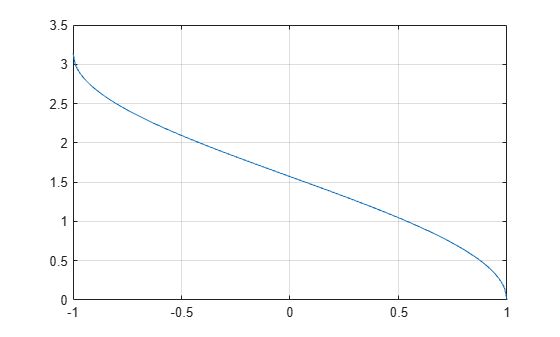acos

Description

example

Y = acos(X) returns the Inverse Cosine (cos-1) of the elements of X in radians. The function accepts both real and complex inputs.

• For real values of X in the interval [-1, 1], acos(X) returns values in the interval [0, π].

• For real values of X outside the interval [-1,1] and for complex values of X, acos(X) returns complex values.

Examples

collapse all

Find the inverse cosine of a value.

y = acos(0)
y = 1.5708

Find the inverse cosine of the elements of vector x. The acos function acts on x element-wise.

x = [0.5i 1+3i -2.2+i];
y = acos(x)
y = 1×3 complex

1.5708 - 0.4812i   1.2632 - 1.8642i   2.6799 - 1.5480i

Plot the inverse cosine function over the intervals $-1\le x\le 1$.

x = -1:.01:1;
plot(x,acos(x))
grid onInput Arguments

collapse all

Cosine of angle, specified as a scalar, vector, matrix, or multidimensional array. The acos operation is element-wise when X is nonscalar.

Data Types: single | double
Complex Number Support: Yes

collapse all

Inverse Cosine

The inverse cosine is defined as

${\mathrm{cos}}^{-1}\left(z\right)=-i\mathrm{log}\left[z+i{\left(1-{z}^{2}\right)}^{1/2}\right].$